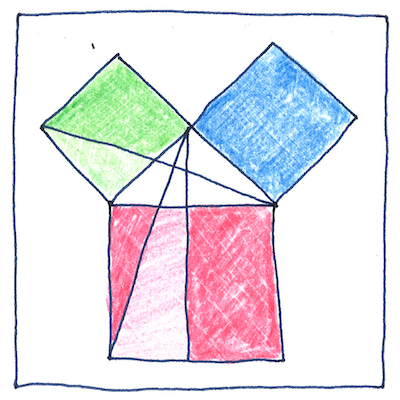# 300 BCE

## The book of science

Tom Sharp

 Euclid geometry

## Geometry

• The character known as Euclid,
• or Eukleidēs in the Greek,
• wrote the textbook on mathematics,
• laying out a system
• based on definitions and axioms,
• each theorem rigorously proven,
• that established the logic
• that all educated people studied
• for twenty-three centuries.

## Mastering

• Euclid took from Plato
• the use of definitions and axioms,
• reworked theorems by Eudoxus of Cnidus,
• perfected theorems by Theaetetus,
• based the books of his Elements
• on Pythagoras of Samos,
• Hippocrates of Chios,
• and other Pythagorean
• or Athenian mathematicians,
• but Euclid worked his borrowings
• into a comprehensive deductive system
• with his own rigorous proofs
• so that today his name is synonymous
• with . . . uh . . . Euclidean geometry.

## Magnitudes

• Euclid measured a line
• only in relation to the length of another line.
• He measured an angle
• only in relation to the perpendicular.
• He used positive numbers and fractions;
• negative numbers were not geometrically meaningful.
• Multiplication of two lengths
• produced an area.
• Numbers were more understandable as geometric quantities
• than as Alexandrian numerals.

## Elements

• Letters (elements in the Greek)
• label points in the diagrams
• so that the text can simply reference them.
1. That the signifier is not the signified dissolves in memory.
2. Etymology persists as meaning.
4. A smell can evoke everything.
• When the magician or comedian points,
• pay attention to the end of his finger.
• (Ha ha. Just kidding!)

Euclidean geometry assumes parallel lines never intersect, which is true of latitudinal lines on a globe, but not true of longitudinal lines. Today we know that all space is curved. Einstein showed that physical space affected by gravity is non-Euclidean.

The first Greek to divide a circle into 360 degrees was Hipparchus.

Alexandrian numerals were similar to Roman numerals, an additive decimal system with values given to letters of the Greek alphabet. The Greek letter Epsilon (Ε) was 5; Nu (Ν) was 50; Phi (Φ) was 500, so that ΦΝΕ (added together) was 555.